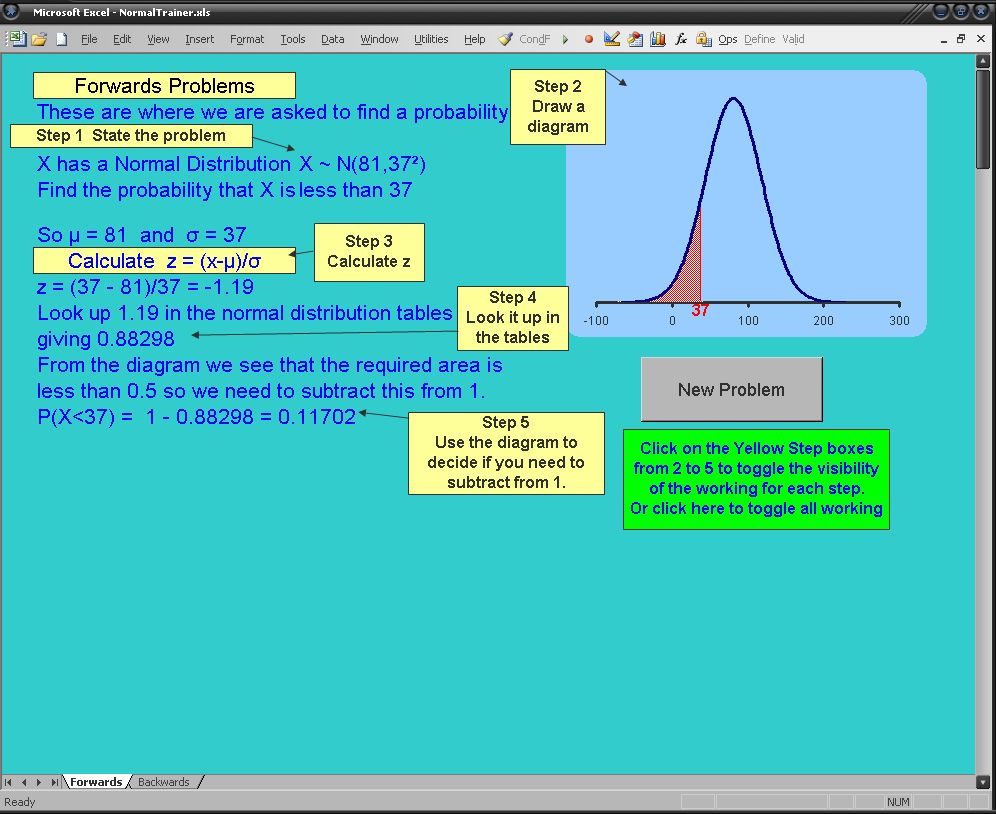# Normal Distribution Trainer

There are two files on MathFiles.com which aim to teach a systematic and efficient approach to solving basic Normal distribution problems.
The method I use is summarised in Normal Distribution Problems pdf
This method can then be demonstrated and practiced using the Normal Trainer Excel file.The Normal Trainer Excel File

First I emphasise the distinction between ‘Forwards’ and ‘Backwards’ problems: A ‘Forwards’ problem is where we are given information and asked to find a probability; a ‘Backwards’ problem is where we are given a probability and asked to find something else: either the mean the standard deviation or a value of X.
For ‘Forwards’ problems the key strategy is to draw a diagram showing the area of the Normal distribution graph which represents the probability we are trying to find. If this area is seen to be greater than 0.5 we simply need to calculate and then look up the z-value in tables and write down the corresponding probability.
If the area is seen to be less than 0.5 we calculate and look up the z-value in tables and take the corresponding probability away from 1. The important thing here is to realise that this strategy will work independently of whether z is positive or negative and hence it simplifies the process considerably.

For ‘Backwards’ problems the key step is to draw a Normal distribution diagram showing the area representing the probability, p, we are given in the question and also the area representing the probability of the opposite event (1-p). We then reverse look up the largest of these two probabilities in the Normal distribution tables to find the z-value and determine if it is positive or negative by using the diagram: if z is on the left it’s negative, if it’s on the right it’s positive. Finally we put all our information into the standardization formula and solve for the unknown.